## 一、python+OpenCV_06_图像直方图（histogram）HOG

0-255个像素值，每个值出现的频次是多少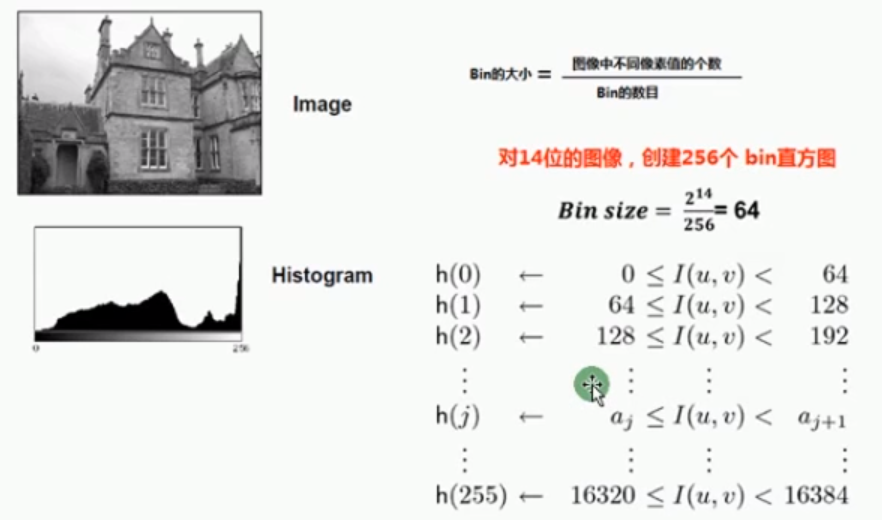256个bin意思就是有256个竖的条条，bin-size是64，意思是以64为bin宽度，统计频次

``````# -*- coding:utf-8 -*-
# Linda Li 2019/8/25 15:44 cv_28_图像直方图 PyCharm

import cv2 as cv
import numpy as np
from matplotlib import pyplot as plt

def plot_demo(image):
"""
image.ravel()统计频次的
bins 256,256条直方
range[0,256]
"""
plt.hist(image.ravel(), 256, [0, 256])
plt.show()

print("-------hello python--------")
cv.namedWindow("input image", cv.WINDOW_AUTOSIZE)
cv.imshow("input image", src)

plot_demo(src)

cv.waitKey(0)
cv.destroyAllWindows()

``````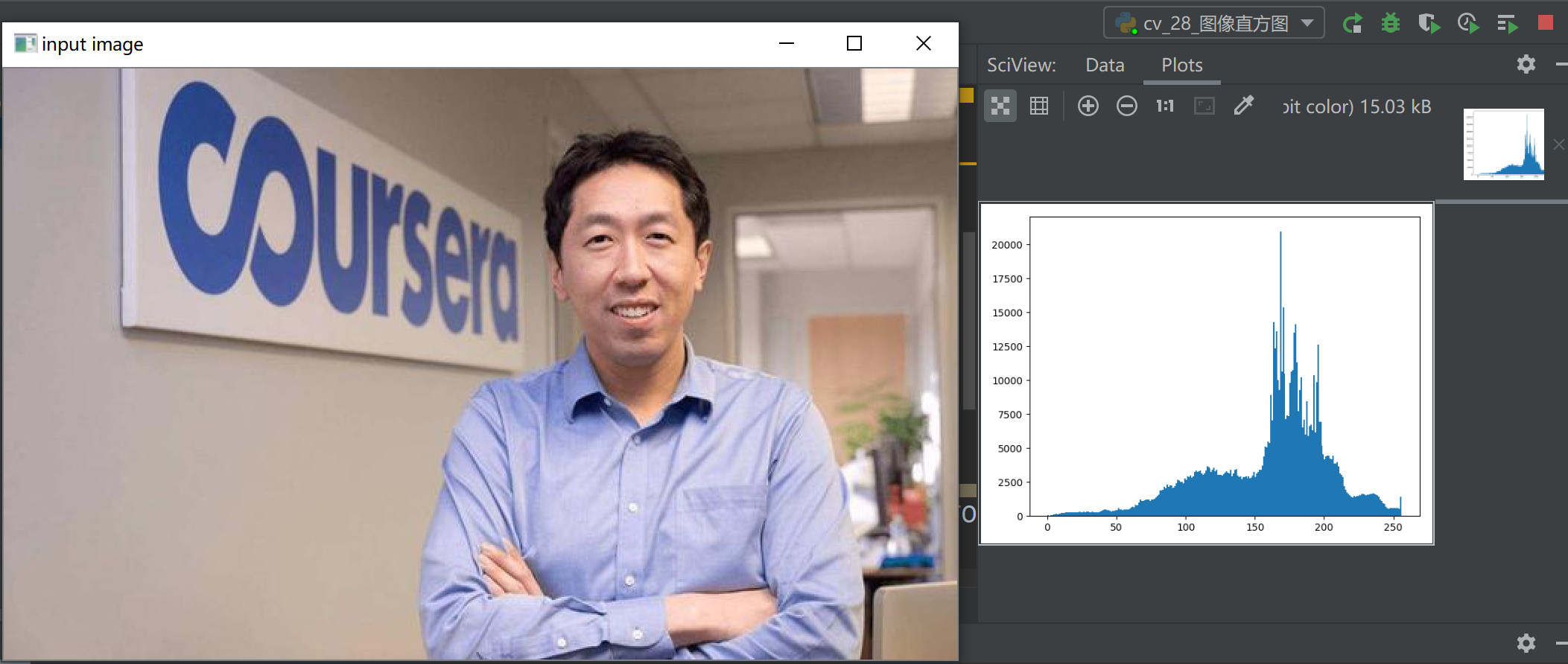## 二、直方图的API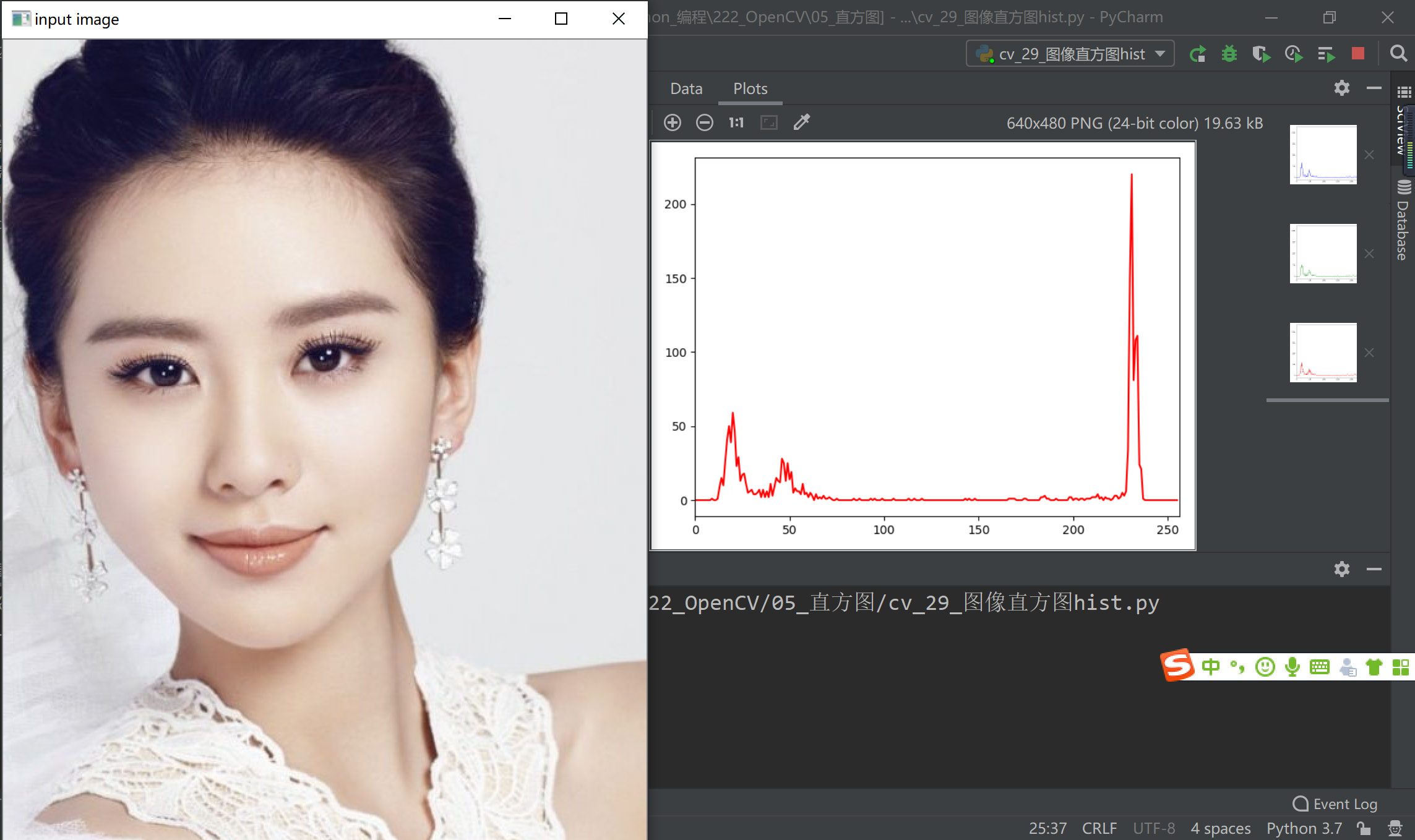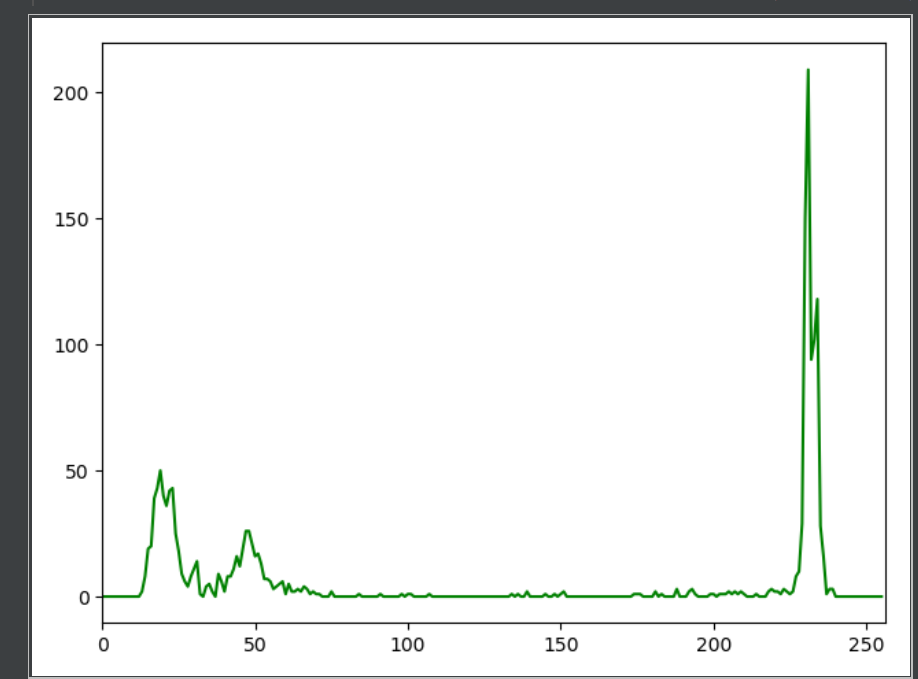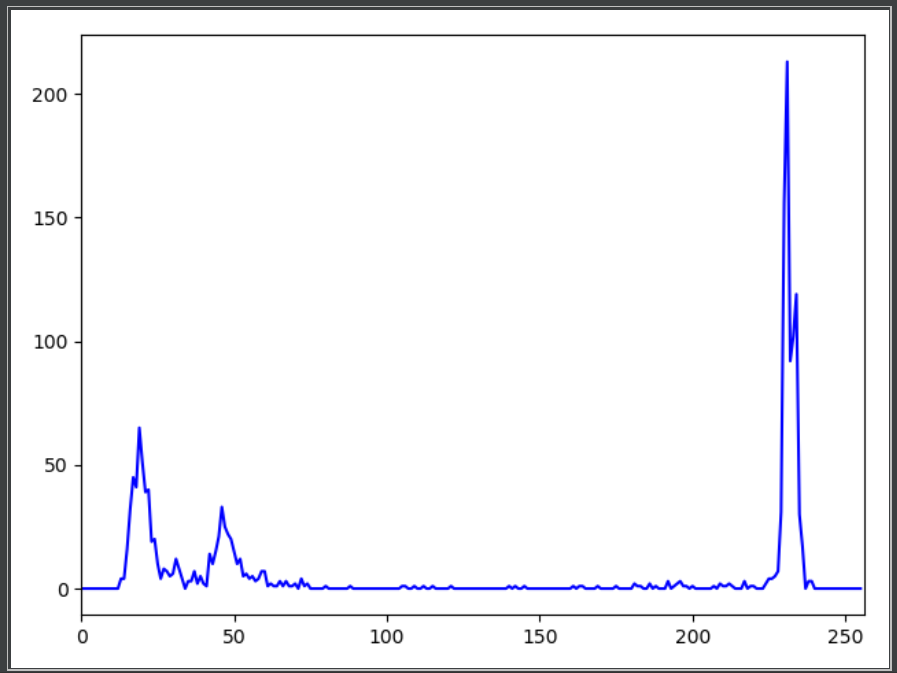整由于张图片比较偏白色，所以三个通道都在255左右取得波峰，把这些值抠出来，其实就可确定人的位置，背景和前景

``````# -*- coding:utf-8 -*-
# Linda Li 2019/8/25 15:44 cv_28_图像直方图 PyCharm

import cv2 as cv
import numpy as np
from matplotlib import pyplot as plt

def plot_hist(image):
"""绘制三个通道的图像，这个是个第三方的API模板"""
# 三个颜色 i = 0, 1, 2
color = ('blue', 'green', 'red')

# 绘制每一个颜色对应的直方图
for i, color in enumerate(color):

# 计算直方图
hist = cv.calcHist(image, [i], None, , [0, 256])
plt.plot(hist, color=color)
plt.xlim([0, 256])
plt.show()

print("-------hello python--------")
cv.namedWindow("input image", cv.WINDOW_AUTOSIZE)
cv.imshow("input image", src)

plot_hist(src)

cv.waitKey(0)
cv.destroyAllWindows()
``````

## 三、直方图均值化——增强对比度（全局）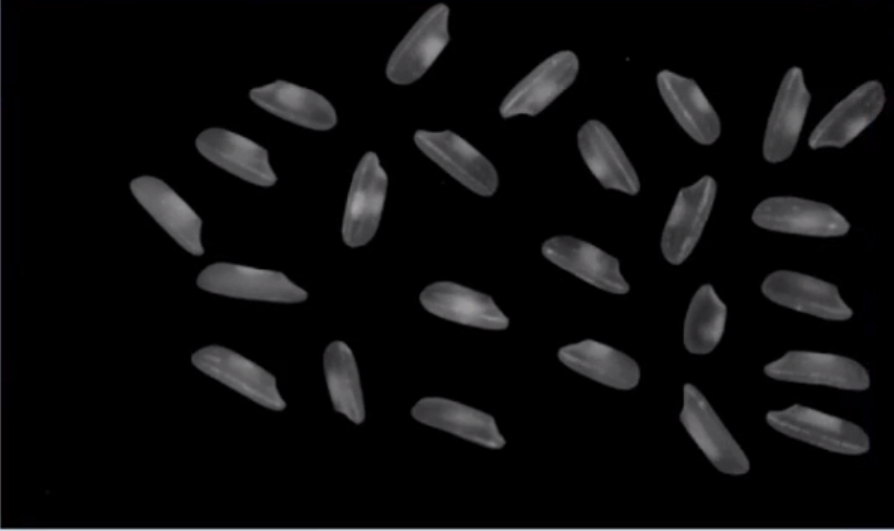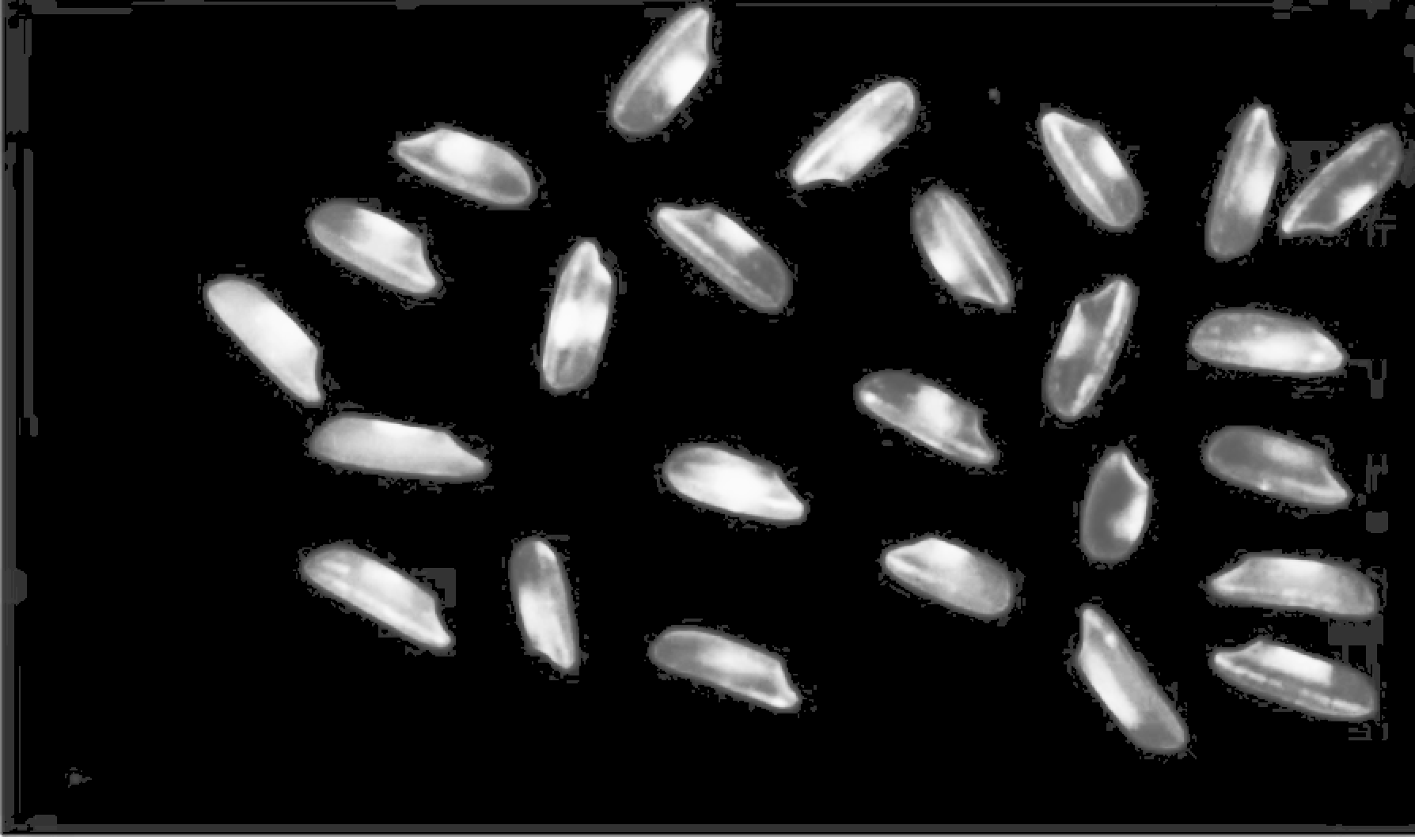``````# -*- coding:utf-8 -*-
# Linda Li 2019/8/25 17:37 cv_30_直方图均衡化 PyCharm

import cv2 as cv
import numpy as np
from matplotlib import pyplot as plt

def equal_hist(image):
"""直方图均值化,是图像增强的一个手段"""

# opencv中的直方图均衡化都是基于灰度图像的，所以要先将图像变成灰度图像
gray = cv.cvtColor(image, cv.COLOR_BGR2GRAY)
dst = cv.equalizeHist(gray)
cv.imshow("equal_hist", dst)

print("-------hello python--------")
cv.namedWindow("input image", cv.WINDOW_AUTOSIZE)
cv.imshow("input image", src)

equal_hist(src)

cv.waitKey(0)
cv.destroyAllWindows()
``````

## 四、直方图均值化——增强对比度（局部）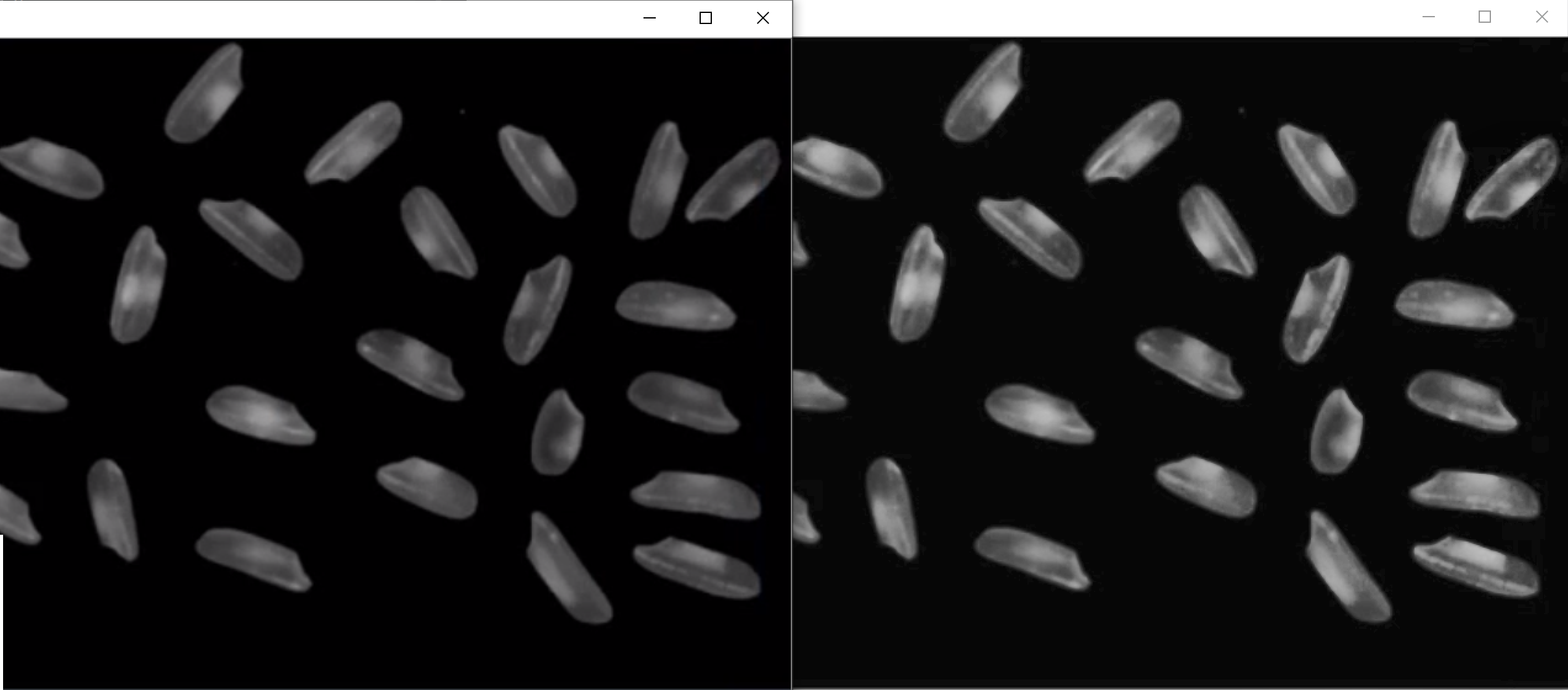``````# -*- coding:utf-8 -*-
# Linda Li 2019/8/25 17:37 cv_30_局部直方图均衡化 PyCharm

import cv2 as cv
import numpy as np
from matplotlib import pyplot as plt

def clahe_demo(image):
"""局部自适应的直方图均衡化"""

# openCv中的直方图均衡化都是基于灰度图像的，所以要先将图像变成灰度图像
gray = cv.cvtColor(image, cv.COLOR_BGR2GRAY)

# clipLimit指的是差异的大小， 8 * 8 的区域
clahe = cv.createCLAHE(clipLimit=5.0, tileGridSize=(8, 8))

dst = clahe.apply(gray)
cv.imshow("clahe_demo", dst)

print("-------hello python--------")
cv.namedWindow("input image", cv.WINDOW_AUTOSIZE)
cv.imshow("input image", src)

clahe_demo(src)

cv.waitKey(0)
cv.destroyAllWindows()
``````

## 五、直方图的比较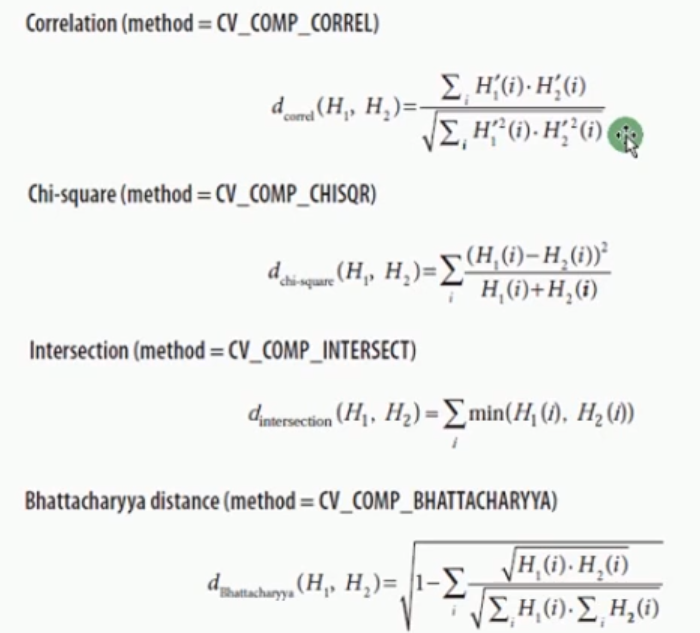1. 相关性 =1
2. 卡方
3. 相交性
4. 巴氏距离
== 两张图片如果相似度很高的话，2,3，4都应该很小==
找相似的图片可以通过做出两张图片的两个直方图排除50%，剩下的可以考虑用比较高级的特征，比如纹理
，边缘，角度，梯度特征再比较``````# -*- coding:utf-8 -*-
# Linda Li 2019/8/25 17:37 cv_30_局部直方图均衡化 PyCharm

import cv2 as cv
import numpy as np
from matplotlib import pyplot as plt

def create_rgb_hist(image):
h, w, c = image.shape

# 初始化1维度
rgb_hist = np.zeros([16*16*16, 1], np.float32)

# bins bin的数量 16
bsize = 256 / 16
for row in range(h):
for col in range(w):
b = image[row, col, 0]
g = image[row, col, 1]
r = image[row, col, 2]

# 把float转换为int之后才可以做index，每一个出现的是在哪个颜色里面，就给他加一
index = np.int(b/bsize) * 16 * 16 + np.int(g/bsize) * 16 + np.int(r/bsize)

# 本来都是0，加上1
rgb_hist[np.int(index), 0] = rgb_hist[np.int(index), 0] + 1

return rgb_hist

def hist_compare(image1, image2):
hist1 = create_rgb_hist(image1)
hist2 = create_rgb_hist(image2)

# 1.巴氏距离
match1 = cv.compareHist(hist1, hist2, cv.HISTCMP_BHATTACHARYYA)

# 2.相关性
match2 = cv.compareHist(hist1, hist2, cv.HISTCMP_CORREL)

# 3.卡方-不太好度量，还是用1,2比较多
match3 = cv.compareHist(hist1, hist2, cv.HISTCMP_CHISQR)

print("巴氏距离：%s, 相关性: %s, 卡方： %s" % (match1, match2, match3))

print("-------hello python--------")
cv.imshow("image1", image1)
cv.imshow("image2", image2)
cv.imshow("image3", image3)
# hist_compare(image1, image2)
hist_compare(image1, image3)

cv.waitKey(0)
cv.destroyAllWindows()
``````

## 六、直方图反向投影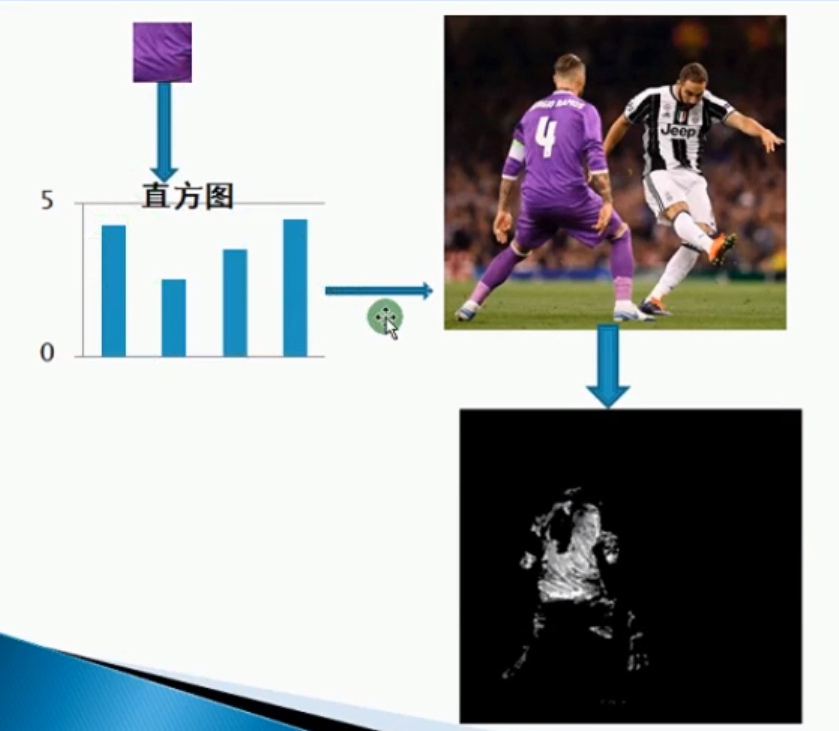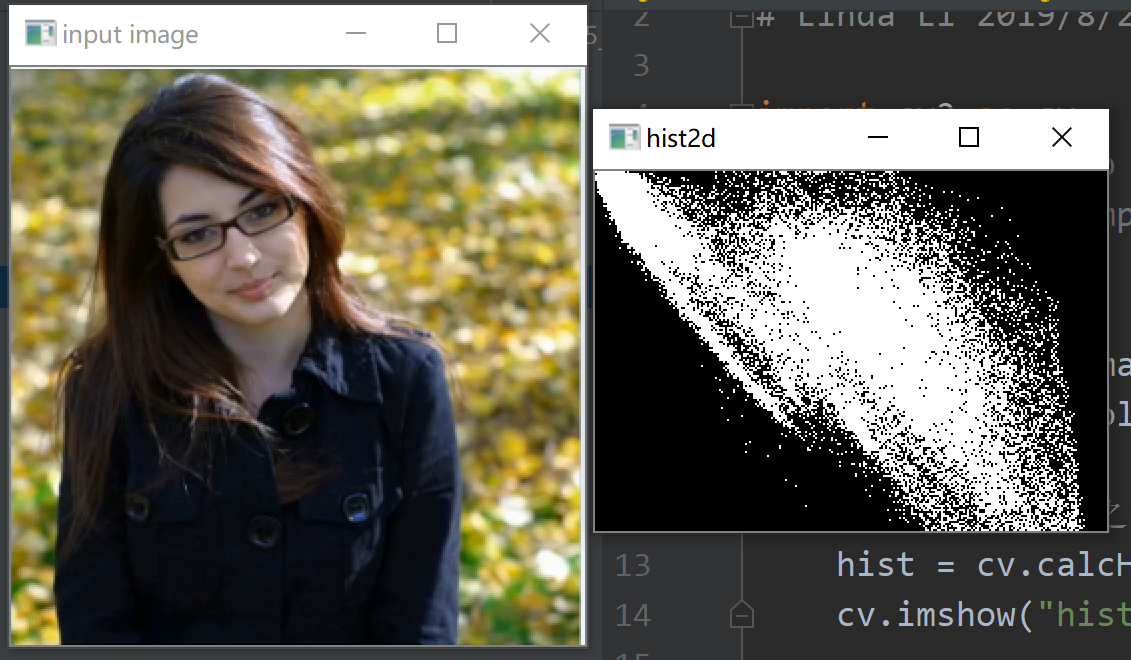### 企业微信怎么输入服务器id,在后台设置企业微信信息时，如何获得企业ID（ CorpId ）和 Secret ？..._weixin_39995943的博客-程序员ITS304

**一、登录企业微信管理后台**点击下方的链接，前往企业微信管理后台，使用管理员的企业微信扫码登录[https://work.weixin.qq.com/](https://work.weixin.qq.com/)**二、获取企业ID**( CorpId )在企业微信管理后台点击“我的企业”，如下图所示。![](https://txc.gtimg.com/data/174823/2020/0706...

### python seaborn_【Python可视化3】Seaborn之箱线图与小提琴图_weixin_39640090的博客-程序员ITS304

Seaborn是基于matplotlib的Python可视化库。它提供了一个高级界面来绘制有吸引力的统计图形。Seaborn其实是在matplotlib的基础上进行了更高级的API封装，从而使得作图更加容易，不需要经过大量的调整就能使你的图变得精致。但应强调的是，应该把Seaborn视为matplotlib的补充，而不是替代物。注：所有代码均在IPython notebook中实现box...

### 学废了吗_后台管理项目必备_码者_荣耀的博客-程序员ITS304

vue2 + elementUI + echarts1.侧边栏菜单选中后刷新页面, 保持状态2.侧边栏折叠/展开后刷新页面, 保持状态 a.将collapse:false保存在vuex的state中, 在页面调用 b.App.vue页面页面刷新时，如何保持原有vuex中的state信息 - 浅笑· - 博客园3.隐藏echarts滑轮两侧的字dataZoom-slider.textStyle. color :"transpare...

### LSA和PLSA_LZXandTM的博客-程序员ITS304_plsa

1.词袋模型   词袋模型(BOW, Bag-of-Words) 模型是NLP领域中的一个基本假设，一个文档(document)被表示为一组单词(word/term)的无序组合，而忽略了语法或者词序的部分,存在缺陷:  稀疏性: 对于大词典，尤其是包括了生僻字的词典，文档稀疏性不可避免；  多义词: BOW模型只统计单词出现的次数，而忽略了一个词可能存在多种含义，一词多义；  同义